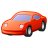Announcements
Applications are open for the 2024 Qlik Luminary Program. Apply by December 15 here.
cancel
Showing results for
Did you mean:Partner - Contributor III

## Getting Values More than equal to Total

Hi Experts,

I have calculated RM% . Now my requirement is based on selection the records which has the value greater than equal to total RM% on visible. For Example

In below image my Avg. RM% is 69.6% and now I want only those records in my table whose RM% Value is greater than equal to  69.6%.

Like from the image 82.6%,71.9%,339.5% and all.

Labels (4)

• ### greater than in measure

15 RepliesMaster II

if let's say your expression is avg(field) then may be if(avg(field)> avg(total field) ,avg(field),null()) .. then suppress zero.

Learning never stops.MVP

What exactly is the expression behind RM%?Partner - Contributor III
Author

(sum(billing qty)*sum(material)) / sum(Billin Price) is the logic for RM%Partner - Contributor III
Author
Its not working, Its giving same avg. RM% value against all values. like 69% against all records.MVP

So, total average should be like this

```Avg(TOTAL Aggr(
(Sum(billing qty)*Sum(material)) / Sum(Billin Price)
, YourChartDimensions))```Partner - Contributor III
Author

Hi Sunny,

I have calculated the avg. now the requirement is on any selection (Year, Month, Any dimension filter) based on RM% I need show only those records which have the RM% value greater than equal to Total Avg. RM% .MVP

Should be just like how @pradosh_thakur  mentioned...

If(

```If(
(Sum(billing qty)*Sum(material)) / Sum(Billin Price) >=
Avg(TOTAL Aggr((Sum(billing qty)*Sum(material)) / Sum(Billin Price), YourChartDimensions)),
(Sum(billing qty)*Sum(material)) / Sum(Billin Price))```Partner - Contributor III
Author

Hi Sunny it's not working for me,

I am calculating RM% using the below expression.

(

sum(
aggr(Sum({<
[Fiscal Year] = {">=\$(=Max([Fiscal Year]))"}
,Procurement = {'E'}
,[Fiscal Month] //= {"\$(=Month(Max(CalendarDate)))"}
,[Fiscal Quarter]
>}[Billing Qty]),PlantCode,[Material No],[Fiscal Month])
*
aggr(Sum({<
[Fiscal Year] = {">=\$(=Max([Fiscal Year]))"}
,[Fiscal Month] //= {"\$(=Month(Max(CalendarDate)))"}
,[Costing Variant] = {'YPC1'}
,[Fiscal Quarter]
>}BOM),PlantCode,[Material No],[Fiscal Month])
)
/
Sum({<
[Fiscal Year] = {">=\$(=Max([Fiscal Year]))"}
,Procurement = {'E'}
//,[Fiscal Month] //= {"\$(=Month(Max(CalendarDate)))"}
,[Fiscal Quarter]
>}[Base Price])

)

If i am using this expression in the way you suggested its not working.Master II

Hi Deepak,

A couple of questions before answering this

1: What is the total function you  have applied on the above mentioned expression, is it auto or average?

2: Do you have  PlantCode,[Material No],[Fiscal Month] as your dimension in the table ? If yes then aggr might not be needed in the case

I would request you to share a sample so we could provide you with a better solution. You are already using aggr 2 times. Like i said without seeing the dimension we can provide you an answer which might slow down your dashboard.

Thanks